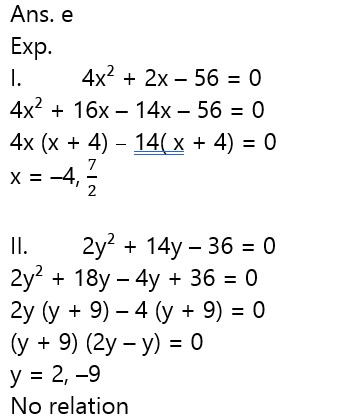# RBI Assistant Pre 2023 Quantitative Aptitude Quiz -1

## RBI Assistant Pre 2023 Quantitative Aptitude

Quantitative Aptitude is the most significant part of almost all competitive exams. Many candidates find difficulty in solving Quantitative Aptitude Questions. The only way to ace this section is to practice as many questions as possible. And, to help you practice more and more questions, we have come up with the RBI Assistant Pre 2023 Quantitative Aptitude Quiz. This RBI Assistant Pre 2023 Quantitative Aptitude Quiz is completely Free. This RBI Assistant Pre 2023 Quantitative Aptitude Quiz includes a variety of questions that are asked in exams. Candidates will be provided with a detailed solution for each question in this RBI Assistant Pre 2023 Quantitative Aptitude Quiz. This RBI Assistant Pre 2023 Quantitative Aptitude Quiz will assist candidates in better preparing for upcoming exams.

Directions (1-5):  Given below is the line graph which shows total number of admission in two schools in the six different years. Read line graph carefully and answer the questions: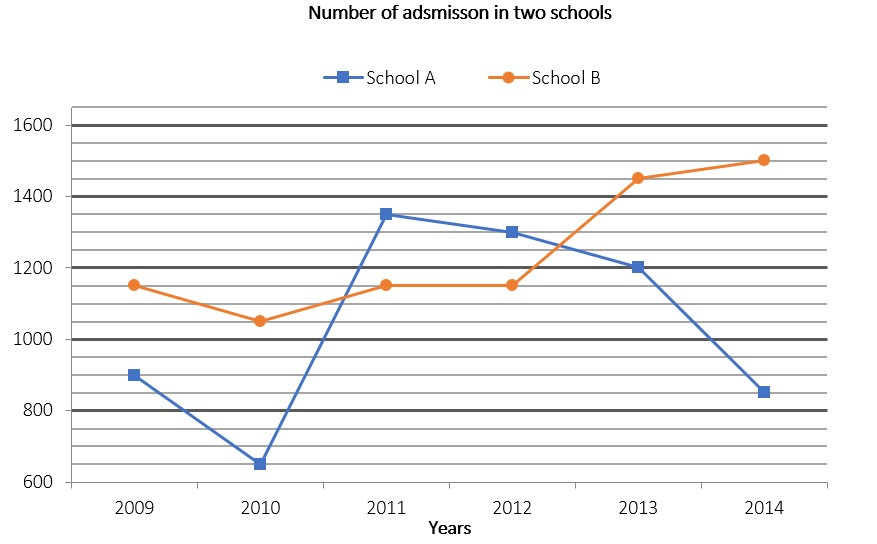1. If 10% and 20% of total students taken admission in school B in 2012 and 2014 respectively have taken admission in 12th class, then find total students who take admission in 12th class in school B in the years 2012 and 2014 together are what percent of total admission taken by school A and B together in year 2011?

(a)  67/5%

(b)  92/5%

(c)  82/5%

(d)  69/5%

(e)  83/5%1. What is the ratio between total admission taken by school A in 2009, 2010 and 2014 together to total admission taken by school B in 2010, 2011 and 2013 together?

(a) 73 : 48

(b) 48 : 73

(c) 46 : 73

(d) 77 : 48

(e) 79 : 48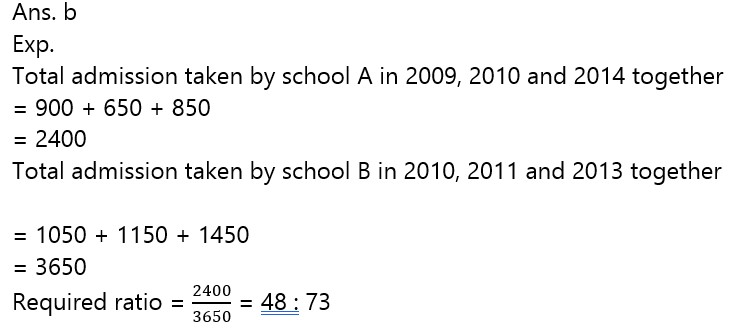1. Number of admission taken by school A in 2009 and 2011 together is what percent more or less than number of admission taken by school B in 2011 and 2013 together?

(a)  198/13%

(b)  175/13%

(c)  227/13%

(d)   253/13%

(e)   149/13%1. If number of admission taken by school A in 2008 is 300% more than difference between number of admission taken by school A and B in 2009, then find average number of admission taken by school A in the years 2008 & 2013?

(a) 1000

(b) 1200

(c) 1300

(d) 1500

(e) 1100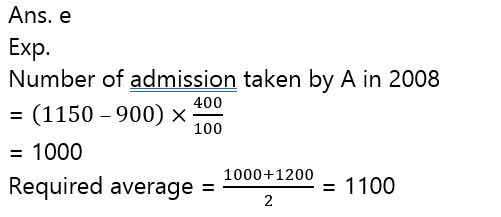1. What is the difference between average number of admission taken by school A in the years 2010, 2011 & 2012 and average numbers of admission taken by school B in the years 2009, 2012 & 2013 ?

(a) 100

(b) 80

(c) 140

(d) 150

(e) 180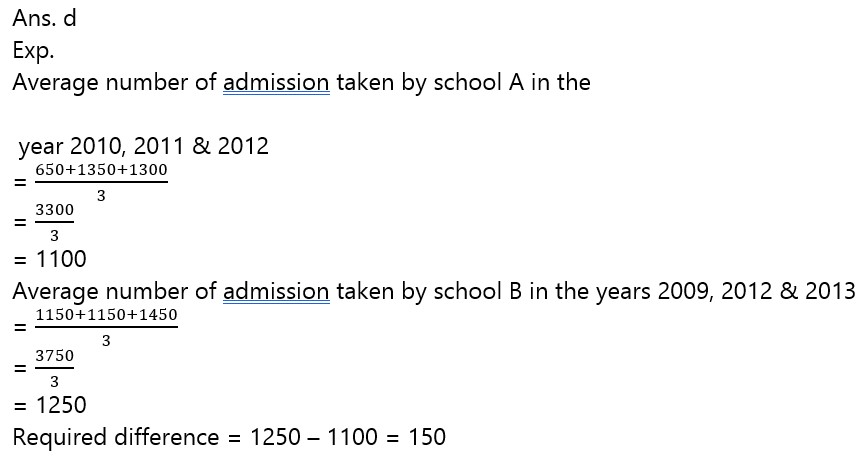Directions (6-10): In each of these questions, two equations (I) and (II) are given. You have to solve both the equations and give answer. between x and y.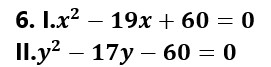(a)if x>y

(b) if x≥y

(c)if x<y

(d)if x ≤y

(e)if x = y or no relation can be established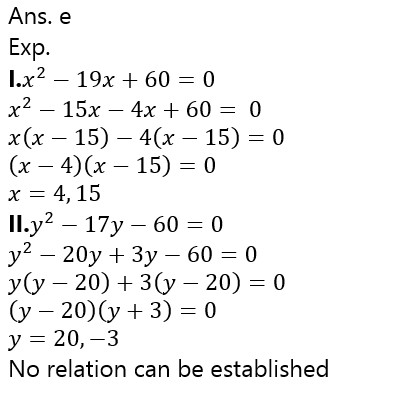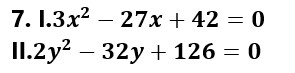(a)if x>y

(b) if x≥y

(c)if x<y

(d)if x ≤y

(e)if x = y or no relation can be established(a)if x>y

(b) if x≥y

(c)if x<y

(d)if x ≤y

(e)if x = y or no relation can be established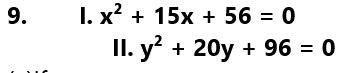(a)if x>y

(b) if x≥y

(c)if x<y

(d)if x ≤y

(e)if x = y or no relation can be established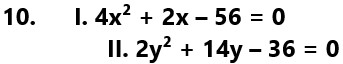(a)if x>y

(b) if x≥y

(c)if x<y

(d)if x ≤y

(e)if x = y or no relation can be established